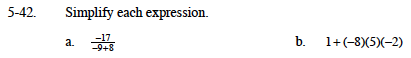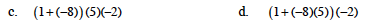### Home > MC1 > Chapter 5 > Lesson 5.2.1 > Problem5-42

5-42.Here, be sure to simplify the denominator (−9 + 8) before dividing the expression.

Adding a positive number to a negative value is similar to taking steps forward when you have already taken several backward. Can you draw a number line to help with this addition?

Try circling the two terms of this expression first. Remember, terms are separated by addition symbols (+).

81This expression has only one term, but part of it is grouped with parentheses. It is important to simplify the expressions inside the parentheses before simplifying the whole expression. Can you find the solution?

70

Notice here that terms are grouped using parentheses.
Be sure to simplify inside of parentheses first.

Within the parentheses, be sure to multiply first and then add, also following the rules you know about negative numbers.